StatLect

Markov Chain Monte Carlo (MCMC) diagnostics

Markov Chain Monte Carlo (MCMC) diagnostics are tools that can be used to check whether the quality of a sample generated with an MCMC algorithm is sufficient to provide an accurate approximation of the target distribution.

In particular, MCMC diagnostics are used to check:

• whether a large portion of the MCMC sample has been drawn from distributions that are significantly different from the target distribution;

• whether the size of the generated sample is too small.Understand the basics of MCMC

Throughout this lecture we are going to assume that you are familiar with the basics of the Markov Chain Monte Carlo method.

Here are some important facts that you need to keep in mind.

An MCMC algorithm produces a sequenceof random variables (or vectors). The sequence has the following properties:

• it is a Markov chain;

• the largeris, the more the distribution ofis similar to the target distribution (in technical terms, we say that the chain converges to its stationary distribution, which is by construction equal to the target distribution);

• in general, two terms of the chainandare not independent. However, the further apart two terms are in the chain, the closer they are to being independent from each other. In other words,andbecome almost independent asgets large.

When we run an MCMC algorithm forperiods, we get an MCMC sample made up of the firstrealizations of the chain: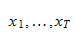and then we use the empirical distribution of the sample to approximate the target distribution.

Understand what can go wrong

What kind of problems do MCMC diagnostics try to spot? Basically, there are two main problems that we may encounter.

Problem 1 - A large portion of the sample is drawn from distributions that are significantly different from the target

In general, the distribution of the starting valueis different from the target distribution. As a consequence, also the distributions of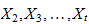are different from the target distribution, although the differences become smaller and smaller asincreases because, by construction, the distribution ofconverges to the target distribution astends to infinity.

It can happen that the chain is slow to converge, that is, after many iterations, the distributions of the draws are still very different from the target distribution. Typically, this happens when the initial valuelies in a region to which the target distribution assigns a very small probability.

When the chain converges slowly, a large portion of our MCMC sample might be made up of observations drawn from distributions that are significantly different from the target distribution.

If we are able to spot this kind of problem, we can try to fix it by:

• discarding a large chunk of initial observations (the so-called burn-in sample);

• increasing the number of iterations (i.e., the sample size).

Both of these fixes have the effect of increasing the proportion of draws extracted from distributions that are (more) similar to the target distribution.

Problem 2 - The effective size of the sample is too small

Remember that, in general, two terms of the chainandare not independent. However, they become almost independent asgets large.

Suppose that we are able to find the smallest numbersuch that, for any,andcan be considered independent for all practical purposes (their degree of dependence is negligible). For the sake of simplicity, also assume that the sample sizeis a multiple of. Then, we can form a sub-sample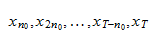of realizations of mutually independent variables. The dimension of this sub-sample, which we call effective sample size, is.

Roughly speaking, the MCMC sample is equivalent to a sample of independent observations having size. The slower the decay of the dependence between the terms of the Markov chain is, the larger, and the smaller the effective sample size is.

When the dependence decays very slowly (is very large), it easily happens that the effective sample size () of the MCMC sample is too small, in the sense that the limited size of the sample makes its empirical distribution a very noisy/imprecise approximation of the target distribution.

If we are able to spot this kind of problem, we can try to fix it by:

• tweaking the MCMC algorithm so as to decrease the dependence (decrease);

• increasing the number of iterations (i.e., the sample size).

Both of these fixes have the effect of increasing the effective sample size.

Note: the definition of effective sample size given here is not rigorous and is different from the definition usually found in MCMC textbooks and papers. Its only purpose is to clearly illustrate the problems that can be generated by slowly decaying dependence.

A fundamental principle behind many MCMC diagnostics

Most MCMC diagnostics test for the absence of Problems 1 and 2 described above.

In particular, absent problems 1 and 2, the following hypotheses hold:

1. the majority of the observations in the MCMC sample have been drawn from distributions that are very similar to the target distribution;

2. the effective size of the sample is not too small.

If these two hypotheses hold, then the implication is that:

By large chunk we mean a sub-sample of adjacent draws representing a significant portion of the overall sample (see Example 1 below).

Many diagnostics are used to test the implication above (call it nice large chunks). If a diagnostic tells us that large chunks are not nice, then our sample is afflicted by either Problem 1 or 2 and its quality is not sufficient.

Sample splits

The simplest way to diagnose problems in an MCMC sample is to split the sample into two or more chunks and check whether we get the same results on all the chunks.

Example Suppose our MCMC sample is made up ofdraws (witheven):where a generic drawis arandom vector. Then, we can divide the sample into two chunks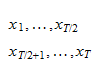and compute their sample means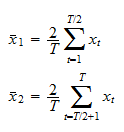If the two sample means are significantly different (we can run a formal statistical test to check the difference), then this is a symptom that the quality of our MCMC sample is not sufficient.

In the previous example, we have compared the sample means of the two sub-samples, but the comparison could be conducted also on other sample moments, for instance, on the sample variances.

The rationale behind this simple diagnostic is very simple. If we find that two chunks of the sample have significantly different empirical distributions, then it must be that at least one of the two is not a good approximation of the target distribution. This contradicts the nice large chunks principle. Therefore, the quality of our sample is not sufficient.

Multiple chains

Another simple way to diagnose problems is to run the MCMC algorithm more than once with different (possibly, very different) starting points, so as to obtain multiple MCMC samples. Then, we check whether we get the same results on all the samples (possibly, after discarding burn-ins). We can conduct the checks by testing whether the sample means or other sample moments are significantly different across the different MCMC samples, similarly to what we did in the previous section (Sample splits).

Trace plots

Trace plots may help us to understand what kind of problem (if any) affects our MCMC sample.

Suppose our MCMC sample is made up ofdrawswhere a generic drawis arandom vector.

A trace plot is a line chart that has:

• time (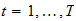) on the x-axis;

• the values taken by one of the coordinates of the draws (e.g.,if the-th coordinate is plotted) on the y-axis.

The next figure contains three trace plots that illustrate typical situations we may encounter.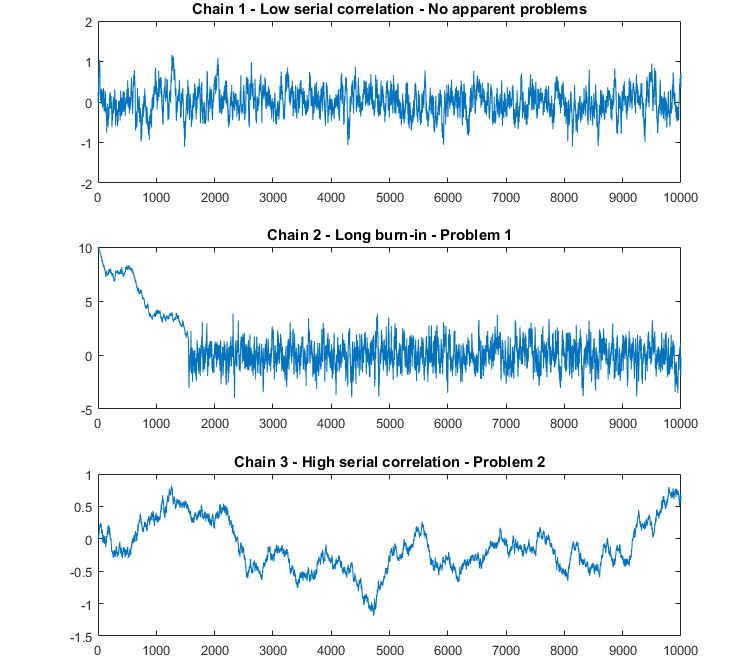In the first trace plot (Chain 1), there are no apparent anomalies. There seems to be a mild serial correlation between successive draws and the chain seems to explore the sample space many times.

In the second plot (Chain 2), the first part of the sample (until around) looks very different from the remaining part. Most likely, the initial distribution and the distributions of the subsequent terms of the chain were very different from the target distribution, but then the chain slowly converged to the target distribution (around). We have Problem 1: a large chunk of the sample is drawn from distributions that are significantly different from the target distribution.

In the third plot (Chain 3), there is a lot of serial correlation between successive draws. The chain is very slow in exploring the sample space. The sample space has been explored only few times. In other words, there seems to be few independent observations in our sample. Quite likely, we have Problem 2: the effective size of our sample is too small.

The next two trace plots show how Problem 1 and 2 can be solved.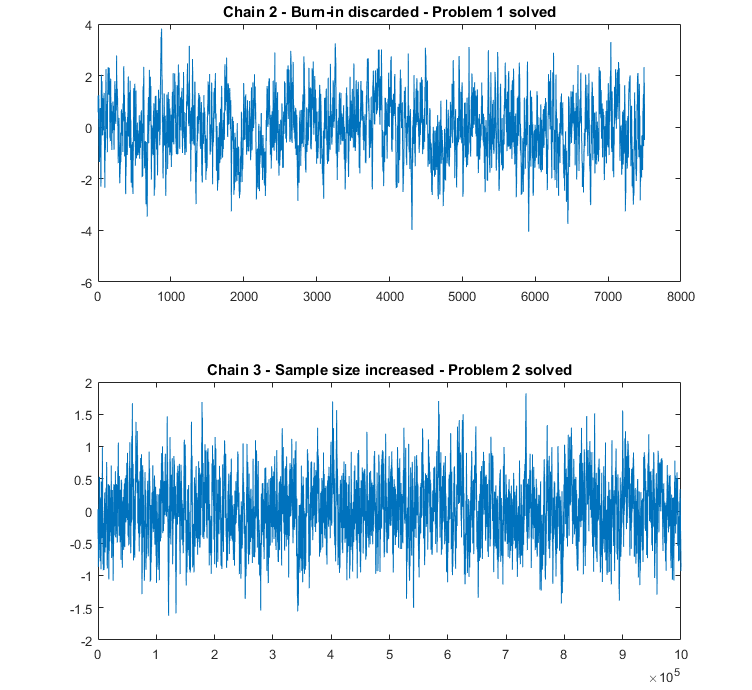In the first plot, the same sample from Chain 2 is used, but the burn-in (the firstobservations) is discarded.

In the second plot, a new sample from Chain 3 is generated, but the sample size is increased dramatically (fromtodraws) so as to increase the effective sample size and let the chain explore the sample space many times.

In both cases, problems seem to have been solved: the trace plots of the MCMC samples do not show any apparent anomaly.

We note that, although trace plots are pretty simple and informal diagnostic tools, they seem to be the most frequently used: if you are trying to publish an MCMC study in a scientific journal, it is very likely that you will be asked to include your trace plots in the study!

Autocorrelation function (ACF) plots

By inspecting trace plots, we can get a sense of the degree of serial correlation of the draws. To measure serial correlation precisely, we can use so-called ACF (autocorrelation function) plots, also called correlograms. From these plots you can see how the sample autocorrelation between the terms of the chain decreases as a function of their lag (see the lecture on autocorrelation for more details).

The next figure contains the sample ACF plots of the three MCMC samples whose trace plots have been discussed in the previous section.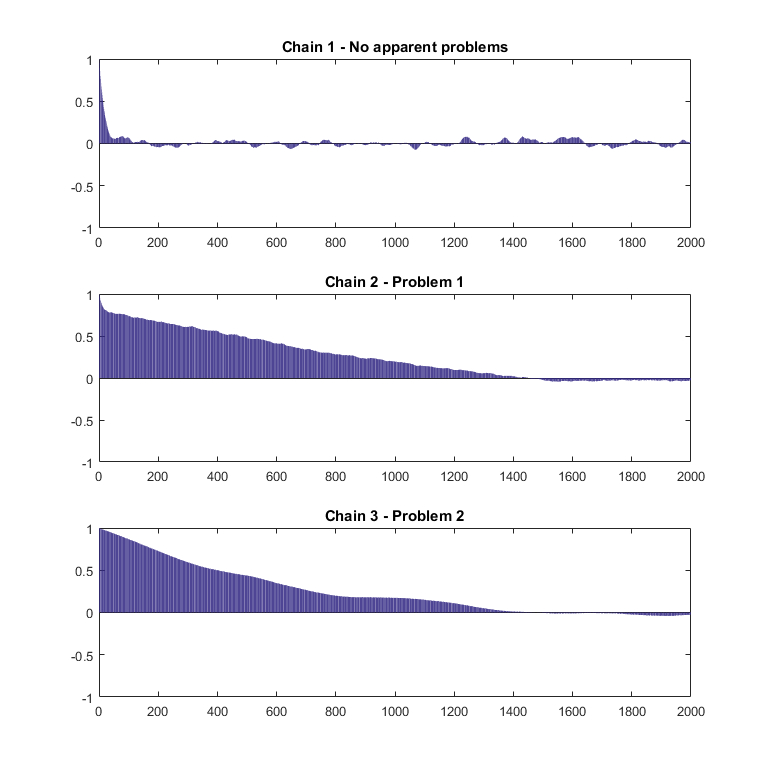The ACF plot of Chain 1 shows that autocorrelation is large at short lags, but then goes to zero pretty quickly (remember that the trace plot did not provide evidence of any problems).

The plots of Chains 2 and 3 show that not only autocorrelation is large at short lags, but it also dies out very slowly. Interestingly the sample ACFs of Chains 2 and 3 are quite similar, even if the trace plots provide evidence that the two chains are affected by different problems (Problems 1 and 2 respectively). According to our experience, this is something that happens quite frequently. Although ACF plots help us to assess the presence of problems and allow us to better quantify autocorrelation, it is difficult to tell from ACFs what the problem is. Therefore, it is advisable to use trace plots and ACF plots together.

Do not rely on a single diagnostic

We should note that MCMC experts generally agree on the following facts:

1. no single diagnostic is perfect;

2. we can never be 100% sure that the quality of an MCMC sample is adequate (diagnostics can help us to spot a problem, but they cannot guarantee the absence of problems).

For these reasons, the best thing we can do is to analyze our MCMC samples as accurately as possible and to employ many different diagnostics to assess their quality.

Run your chains for as long as you can

Having said that performing many diagnostics is always a good idea, we feel like giving another piece of advice: always run your chains for as long as you can, run many chains with different starting points, and do not spare computational resources. This is the best way to avoid problems.

The book

Most of the learning materials found on this website are now available in a traditional textbook format.

Glossary entries
Share